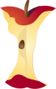The Addition of Four Two Digit NumbersPrintable Worksheets And Lessons

• Step-by-step Lesson- Work on adding various sets of two-digit numbers. We start at pairs and move to 4 in a set.

• Guided Lesson - Two digit horizontal sums, sets of 3 two-digit numbers in vertical addition, count rows and add numbers.

• Guided Lesson Explanation - I walk you through how to approach all these problems.

• Practice Worksheet - Mixed sets of two-digit numbers in a vertical format.

• Matching Worksheet - Match the horizontal two-digit equations to their sum.(Click Here to Upgrade)

Homework Sheets

We start with minimum carrying and then we move to all carrying.

Practice Worksheets

It is tough for students at this level to organize these problems.

Math Skill Quizzes

You might find these to be a bit more fair, skill wise, than the practice sheets.

 Quiz 1 Quiz 2 Quiz 3